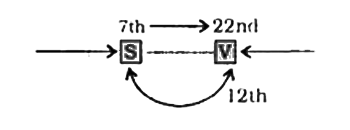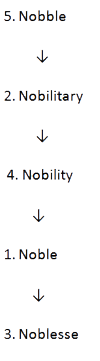# Genpact Reasoning Question

DIRECTIONS for questions (1-2):What should come in place of question mark (?) in the following number/alphabetic series?
1. 3, 4, 7, 11, 18, 29, ?
1. 31
2. 39
3. 43
4. 47
<3 + 1 = 4;
3 + 4 = 7;
4 + 7 = 11;
7 + 11 = 18
11 + 18 = 29;
2. AGMSY, CIOUA, EKQWC, __?___ IOUAG, KQWCI
1. GMSYE
2. FMSYE
3. GNSYD
4. FMYES1. Ashok's mother was 3 times as old as Ashok 5 years ago. After 5 years she will be twice as old as Ashok. How old is Ashok today?
1. 10 years
2. 15 years
3. 20 years
4. 25 years
ago
3(x-5) = (y-5)
⇒ 3x - 15 = y - 5
⇒ 3x - y = 10 ....(i)
5 years hence,
2 (x + 5) = (y + 5)
⇒ 2x + 10 = y + 5
⇒ 2x - y = -5 ....(ii)
From equations (i) and (ii)
2. M is the son of P. Q is the grand¬ daughter of O who is the husband of P. How is M related to O?
1. Son
2. Daughter
3. Mother
4. Father
O is the husband of P. M is the son of P. Therefore, M is the son of O.
3. In a row of boys, Srinath is 7th from the left and Venkat is 12th from the right. If they interchange their positions, Srinath becomes 22nd from the left. How many boys are there in the row?
1. 19
2. 31
3. 33
4. 34Total number of boys in the row = 22 +12 - 1 = 33
DIRECTIONS for question 6 : Arrange the following words as per order in the dictionary.
4. 1. Noble 2. Nobilitary 3. Noblesse 4. Nobility 5. Nobble
1. 1,4,3,2,5
2. 3,4,1,2,5
3. 5,2,4,1,3
4. 2,4,3,5,1
Arrangement of words as per dictionary:5. Which one set of letters when sequentially placed at the gaps in the given letter series shall complete it?
an_nn__ana__na__nan_a
1. annan
2. aanan
3. nanna
4. nana
an [a] / nn [a] / ana / [n] na / [a] na / n [n] a
DIRECTIONS for questions 8 to 10: In each of the following questions, select the related letters/word/number from the given alternatives.
6. Procession : Route : : Earth : ?
1. Space
2. Sun
3. Orbit
4. Highway
Procession proceeds on a certain route. Similarly, Earth revolves around the Sun in its orbit.
7. FERTILIZER : CROPS : : ?
1. TEACHER; EDUCATION
2. CHLORINE: WATER
3. TONIC : BODY
4. PESTICIDE: RATS